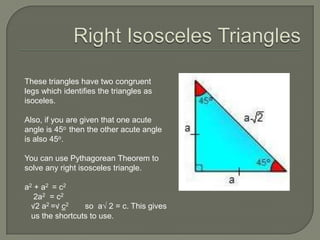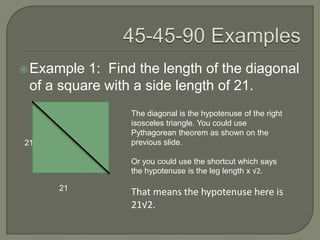Diese Präsentation wurde erfolgreich gemeldet.

# Special right triangles×

1 von 8
1 von 8

# Special right triangles

### Special right triangles

1. 1. NCVPS
2. 2. These triangles have two congruent legs which identifies the triangles as isoceles. Also, if you are given that one acute angle is 45o then the other acute angle is also 45o. You can use Pythagorean Theorem to solve any right isosceles triangle. a2 + a2 = c2 2a2 = c2 √2 a2 =√ c2 so a√ 2 = c. This gives us the shortcuts to use.
3. 3.  Example 1: Find the length of the diagonal of a square with a side length of 21. The diagonal is the hypotenuse of the right isosceles triangle. You could use Pythagorean theorem as shown on the 21 previous slide. Or you could use the shortcut which says the hypotenuse is the leg length x √2. 21 That means the hypotenuse here is 21√2.
4. 4.  Example 2: Given the hypotenuse of a right isosceles triangle is 14, find the length of one leg. You can solve this with Pythagorean theorem: a2 + a2 = 142 2a2 = 196 a2 = 98 14  a = √98 or 7√2  a  a
5. 5. The 30-60-90 triangles also have a short-cut set of relationships. The shortest side is opposite the 300 angle. That side length is denoted “a”. Then the hypotenuse is double of the short leg, so it’s length is 2a. The longer leg is a√3 in length. We know this works because we can verify it by Pythagorean theorem: a2 + (a√3)2 = (2a)2 a2 + 3a2 = 4a2 4a2 = 4a2
6. 6.  Example 3: Find the lengths of the other two sides of the triangle if the x short leg is 7 units. 7 30o y The x represents the hypotenuse so it is double of the short side, or 14 units. The y represents the long leg, so its length is 7√3 units.
7. 7.  Example 4: Find the lengths of the other two sides of the triangle if the 20 hypotenuse is 20 units. x 30o y The x represents the short leg which is half of the hypotenuse, so it is 10 units. The y represents the long leg, so its length is 10√3 units.
8. 8.  Example 5: Find the lengths of the other two sides of the triangle if the y long leg is 15 units. x 30o 15 The easiest way to solve this problem is Pythagorean theorem. What we know is that y = 2x because the hypotenuse is twice as long as the short leg. X2 + 152 = (2x)2 x2 + 225 = 4x2 225 = 3x2 75 = x2 5√3 = x so y = 10√3